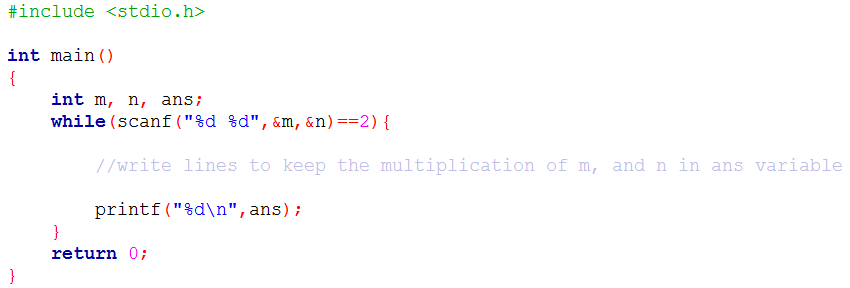Problems
10041 - Sum

Sum

Time Limit: 1 sec

The Problem

In this problem, you will be given 2 integer numbers m, n as input. Your job is to print the summation of m and n.

Your code might look like below:The Input

There are several lines of input in the input file. Each line contains two integer numbers m and n (-100<m,n<100). Input is terminated by EOF.

The Output

For each line of input you have to print a line of output, this line contains an integer which is the summation of m and n.

Sample Input

2 10

6 -3

Sample Output

12

3

Online Practice Contest 1 [School & College Level]

Problem Setter: Shahin ShamS